UNIVERSITY OF WESTERN MACEDONIA FACULTY OF ENGINEERING DEPARTMENT OF ELECTRICAL AND COMPUTER ENGINEERINGHomepage of Theodoros Zygiridis

 The main research interests are within the area of applied engineering mathematics, focusing on computational electromagnetics. Emphasis is given on:  -  finite-difference time-domain (FDTD) methods,  -  high-order algorithms,  -  error-controlling techniques,  -  uncertainty quantification,  -  various applications, such as antennas, bioelectromagnetic problems, WLAN characterization, vehicular simulations, etc.   You may also check the following pages for pertinent publications: Google Scholar ResearchGate

 GPU implementation of high-order FDTD algorithms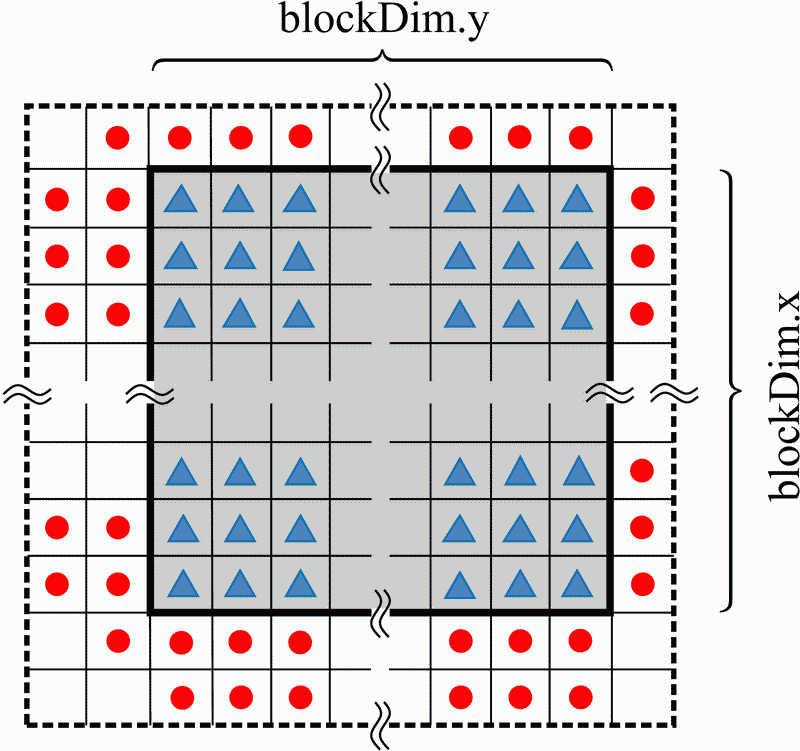A block's access pattern of GPU's shared memory in 2D. Performance of 3D high-order FDTD implementations. The increased efficiency of optimized FDTD schemes can be further improved with the aid of modern GPU computing. Taking advantage of the high number of processing cores featured by current GPUs, we have developed parallelized implementations of high-order discretization schemes. The proposed implementations are characterized by significant speedups, compared to serialized CPU approaches, and can offer minimized computing times for realistic problems.

 Least-squares leapfrog integrators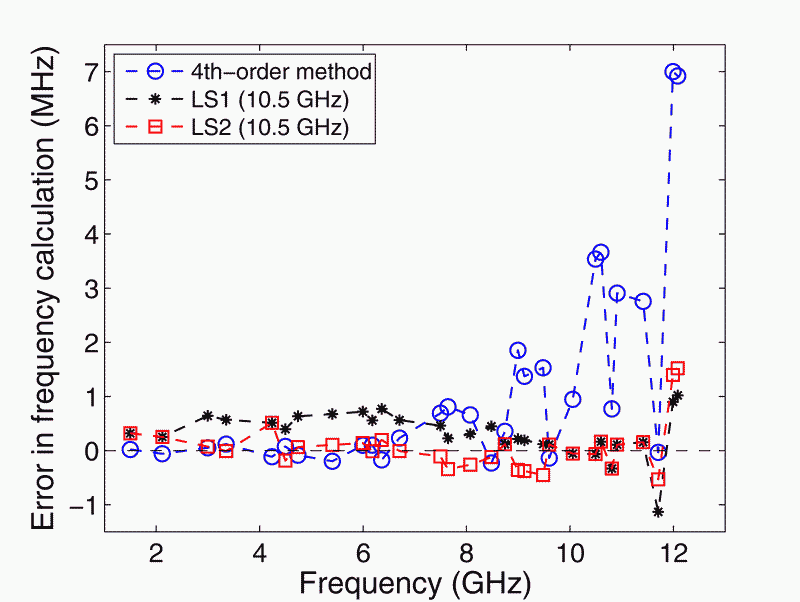Performance of 2D integrators in a mode-detection simulation.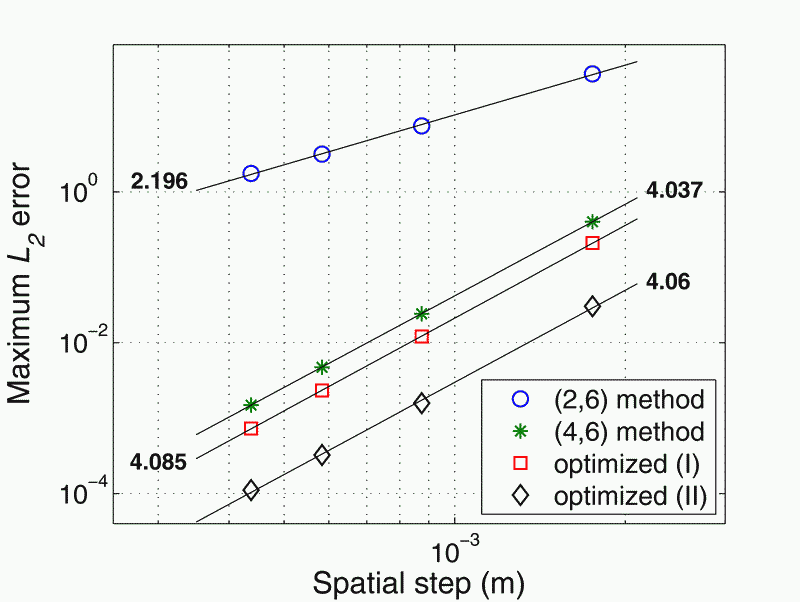Performance of 3D integrators in a single-mode propagation problem. We have applied dispersion-relation-preserving concepts to the construction of optimized leapfrog integration schemes, for Maxwell's equations in 2D and 3D. The spatial part of the fourth-order integrators is replaced by parametric expressions, which are determined via a least-squares error reduction, irrespective of propagation direction. The proposed schemes can be combined with either standard or optimized spatial approximations, for the construction of precise discrete models of electromagnetic problems.

 Bandwidth control of various FDTD schemes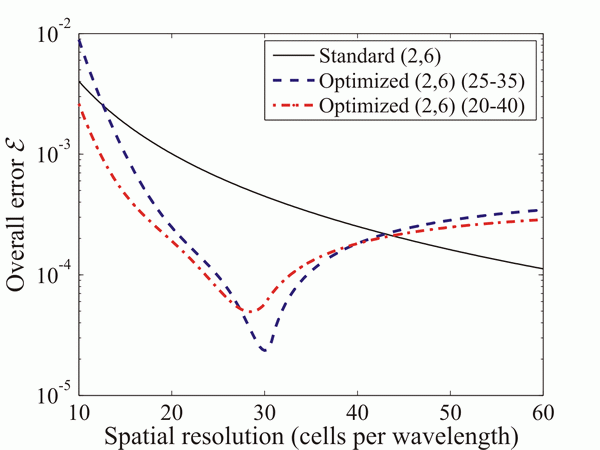Error of standard and proposed (2, 6) schemes (i.e. six-point spatial stencils are used).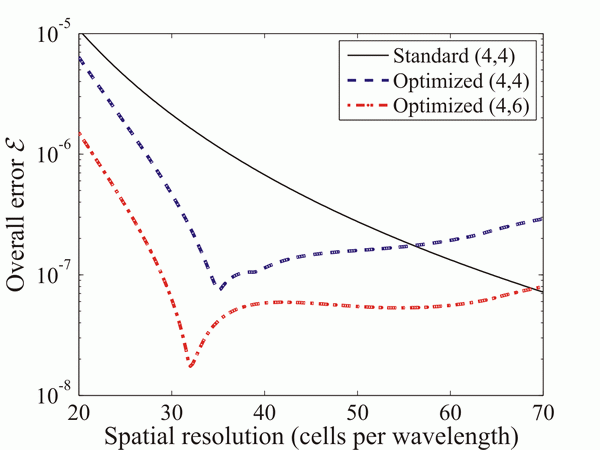Error of proposed (4, N) schemes (i.e. modified time integration similar to the 4-th order leapfrog approach is used). We have presented a systematic methodology to facilitate performance control of several FDTD approaches over a prescribed range of frequencies. The optimization procedure is based on the solution of an overdetermined system of equations with the least-squares method. FDTD schemes with (2, N) structure have been rendered efficient in this way, even when operated close to their stability limits. High-order convergence has been exhibited by optimized techniques with multi-dimensional spatial operators ((4, N) schemes) in broadband problems. The optimized operators suppress dispersion errors in a wideband manner, without practically producing extra computational burden.

 Low-dispersion (2, 4) FDTD method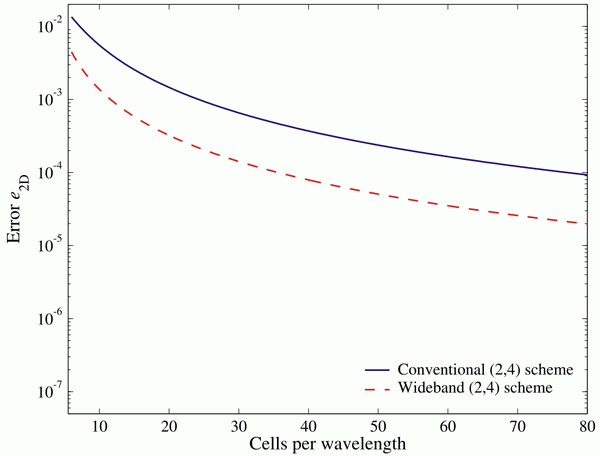Overall error versus grid resolution for the standard and wideband (2, 4) schemes, when maximum time steps are applied. When the standard (2, 4) FDTD scheme is applied using the maximum allowable time step, the obtained performance is practically the same as (or even worse than) Yee's algorithm. This is due to the domination of the temporal error over the (significantly smaller) spatial one. We have decised a methodology that matches spatial and temporal terms of equal order in the numerical dispersion relation, so that modified spatial expressions depending on the time-step size are obtained. The new (2, 4) algorithm outperforms the standard one over all frequencies of practical interest, leading to more efficient discretization scheme. It is thus verified that Taylor-based finite-difference approximations do not necessarily produce the lowest error levels, and the introduction of error-controlling concepts in the design of computational algorithms may ensure performance upgrade.

 FDTD simulations for vehicle communications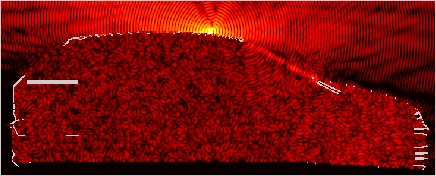Distribution of electric-field intensity at 5.9 GHz, in the case of an antenna mounted on the car's rooftop. In the context of contemporary vehicle-to-vehicle communications, we have simulated the electromagnetic-field interactions of an antenna operating within a realistic car model, at a frequency of 5.9 GHz, for various selections of antenna placements. The required computational lattice of the FDTD method comprised 395x869x269 cells, and the Liao absorbing boundary condition was applied for grid termination.

 3-D optimized FDTD schemes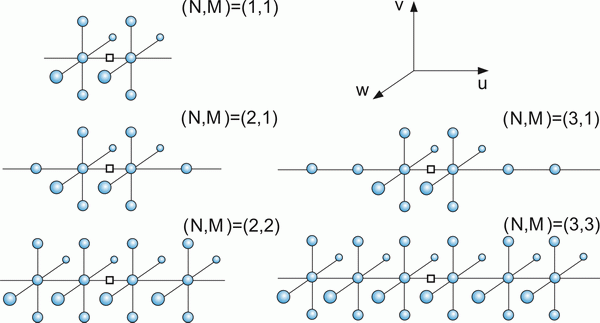Geometry of spatial operators introduced by the optimized FDTD schemes.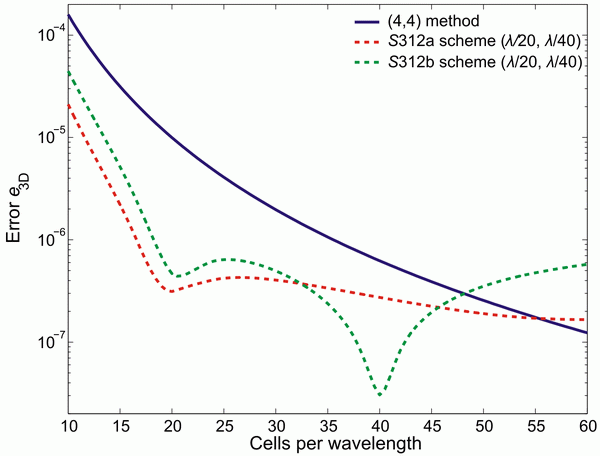Wideband performance of two proposed techniques and comparison to the standard fourth-order method. We have applied dispersion-relation-preserving concepts in order to derive optimized 3-D FDTD discretizations for Maxwell's equations on Cartesian grids. In essence, optimized behavior has been ensured for various spatial stencil sizes and shapes by using a consistent error formula, which is expanded in spherical harmonics. In this manner, overall error control is facilitated, while minimized anisotropy errors can be ensured. In addition, the initial selected stencil is not altered by our methodology, as only frequency-independent coefficients are calculated. We have shown that multi-dimensional definitions of spatial operators can provide improved wideband performance, even when combined with second-order leapfrog updating.

 Numerical error estimation and perfomance control in lossy media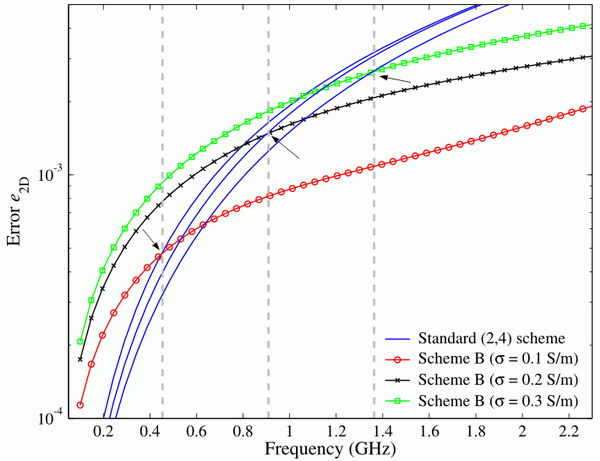Error versus frequency for a lossy medium. The gray lines indicate the theoretically spredicted frequencies where the errors of the standard and proposed methods are expected to coincide. Although various optimized FDTD schemes for lossless media can be found in the pertinent literature, the problem of accuracy improvement in the case of lossy spaces has not received similar attention, perhaps because it appears to be a more complex task. Taking this situation into consideration, a theoretical framework that leads to a new set of FDTD algorithms for problems with electrically-conducting materials has been proposed. The new method efficiently exploits error-minimization concepts and guarantees improved performance over extended parts of the frequency spectrum. One may consider the standard (2,4) method as a complementary member of this family, since it can be derived from the presented procedure as well. We have also verified that valid predictions regarding each scheme’s dynamic range can be made, thanks to the adopted algorithmic foundation.

 High-order discretization of dielectric material interfaces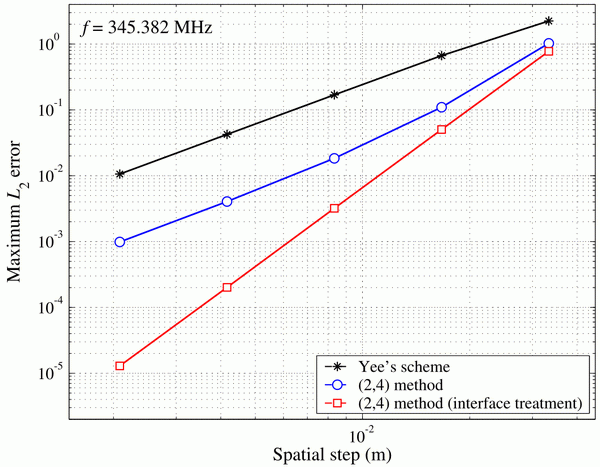Maximum L2 error verus spatial resolution in a partially-filled cavity problem. Conventional finite-difference approximations practically fail when discretization of material interfaces is required, as in this case field components are not as smooth as the considered Taylor-series expansion requires. The discontinuities of the material parameters introduce significant diffculties in the modelling process, especially in the case of high-order discretizations, as trivial approaches unavoidably degrade the convergence of the algorithm. To deal with this problem, we have derived modified finite-difference operators with extended stencils, which are applied only within a transition layer adjacent to the interface, while standard expressions are maintained within the rest of the computational domain. Simulations with a benchmark problem show that high-order convergence rates are recovered, thus verifying the consistent modelling of the interface.

 Error reduction via background material correction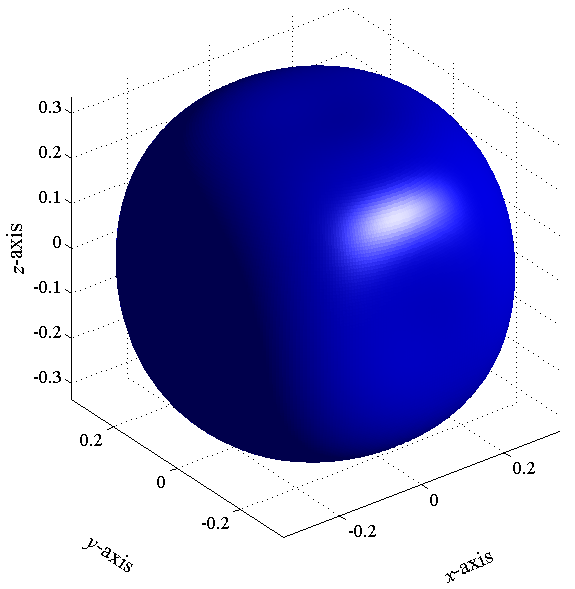Error in the numerical phase velocity of the standard (2, 4) FDTD method, when maximum time step is selected.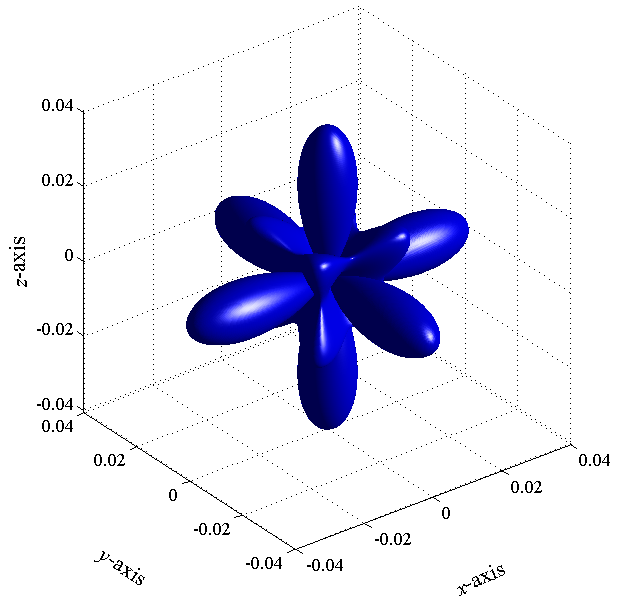Error in the numerical phase velocity of the (2, 4) FDTD method, after material correction has been applied. A simple-to-implement, as well as quite efficient approach for the accuracy improvement of FDTD algorithms is based on the incorporation of slightly modified background media. The idea is to introduce low, yet artificial, dispersion and anisotropy effects that compensate for the numerically induced ones. The design of the new materials is based on single-frequency selection, yet satisfactory wideband performance can be ensured, by careful selection of the design point.

## WLAN computational models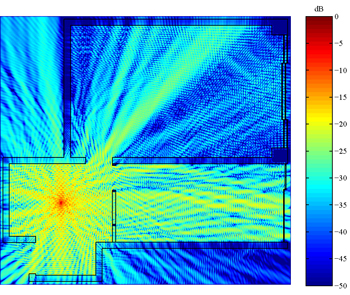Normalized electric-field intensity at 2.44 GHz.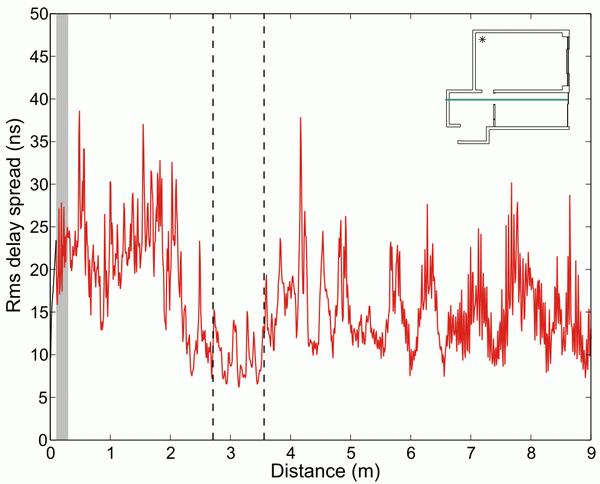Calculation of rms delay spread along a specific route (green line).

The classic FDTD method has been applied for the simulation of indoor wave propagation due to Wireless Local Area Network operation at various frequencies (up to 5.8 GHz). The utilized 2D grid consisted of 1842 x 1710 cells. Results regarding received power levels, small-scale fading and time dispersion were obtained, and compared with statistical models.

 Human exposure to microwaves - Electromagnetic and thermal simulations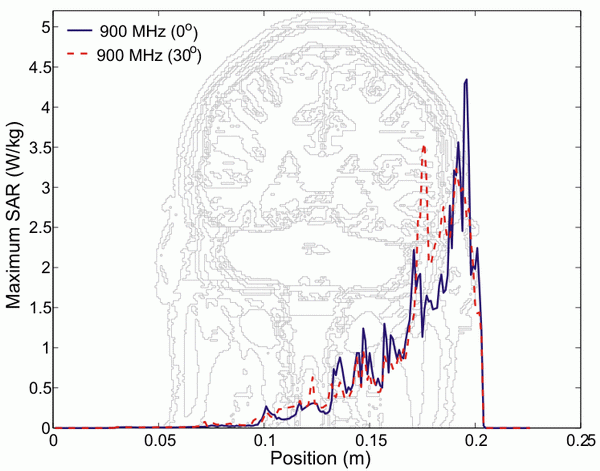Maximum SAR values for two orientations of the mobile phone, operating at 900 MHz.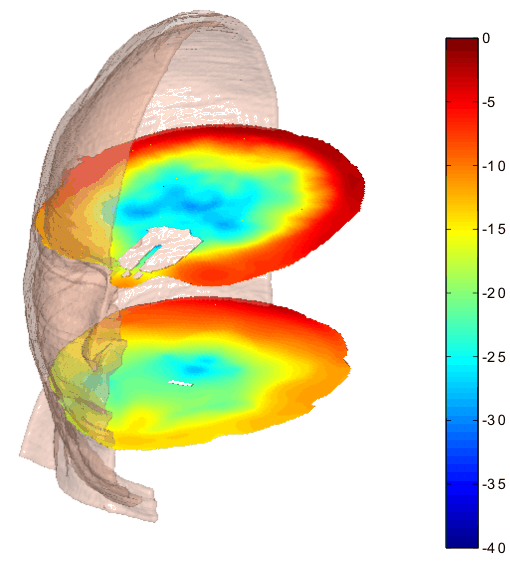Normalized temperature distribution for plane-wave illumination at 2.44 GHz. Another application of the FDTD method was concerned with a numerical study of human exposure to radio-frequency EM fields. Cases involving near-field as well as far-field exposure were examined. A head model was reconstructed from slices including two-dimensional MRI data, which comprised 24 different types of tissue. The overall size of the computational domain was 228 x 272 x 301 cubic Yee cells with 1-mm sides. In addition to the Specific Absorption Rate (SAR), the induced temprerature changes were estimated via the numerical solution of the bioheat equation.

 High-order vector finite element simulations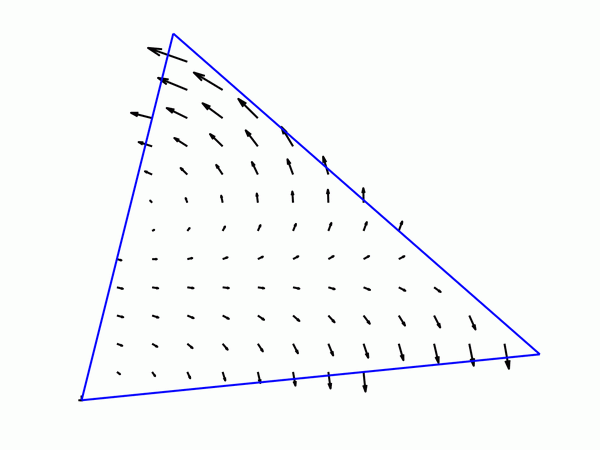High-order edge-related basis function in 2D.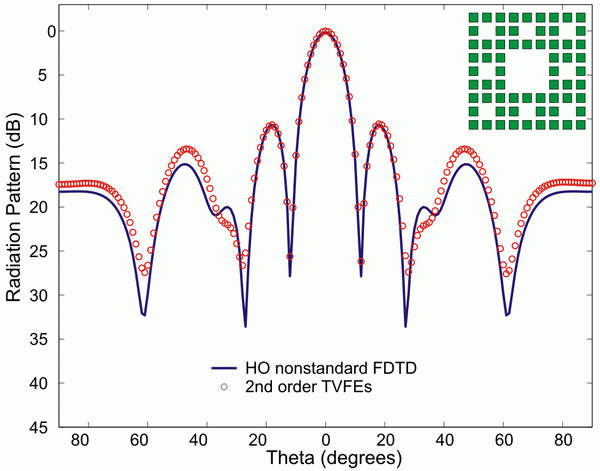Radiation pattern of a 9 x 9 Sierpinski carpet fractal array comprising patch antenna elements. We have applied 3D second-order tangential vector finite elements, combined with a technique that optimizes the convergence of the system solver, to study the radiation characteristics of various standard and fractal antenna arrays of patch elements. Results have been compared with those obtained with a nonstandard FDTD version.